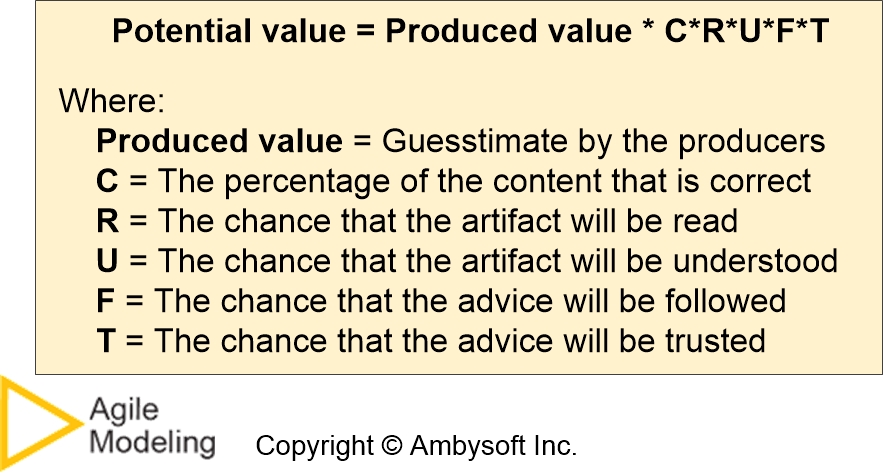# Calculating the Value of Documentation

How do you calculate the value of a document, or in general, the value of an artifact? The challenge is that value is invariably determined by the customer, not the producer. What I mean by this is that you can create the perfect artifact, what you believe to be highly valuable, but if this produced value isn’t taken advantage of then it is never realized.

The potential value of an artifact is calculated with the following formula:

Potential value = Produced value * C * R * U * F * T

where the following factors are measured as follows:

• Produced value is guesstimated by the producers of the artifact.
• C = The percentage of the artifact that is currently “correct”.
• R = The chance that the artifact will be read by the intended audience.
• U = The chance that the document that is actually understood by the intended audience.
• F = The chance that the advice contained in artifact will be followed.
• T = The chance that the advice will be trusted.

The idea is that the CRUFT factors reflect qualitative quality issues for the customers of the artifact. When quality decreases, the value for the customer decreases.

I say potential value rather than realized value, or simply value, to reflect that fact that the formula is based on guesses. The value for produced value is guesstimated by the producer(s) of the artifact and the CRUFT values are also guesses.

## 1. Isn’t Cruft a Bad Thing?

Yes it is. “Cruft” is a slang term which refers to areas of something which are badly designed, poorly implemented, or redundant. It is important to note that cruft is based in part on the perception that someone has of an artifact in addition to the actual quality of that artifact. With respect to documentation, cruft occurs when out of date, redundant, out-of-scope, or poorly written information is included.

## 2. Why is CRUFT Used in the Formula Then?

It’s a matter of history. The original CRUFT formula was published in Dr. Dobbs Journal in 2007. The goal at the time was to show how to calculate the quality of an artifact, rather than the value.

## 3. Summary: Calculating the Value of a Document